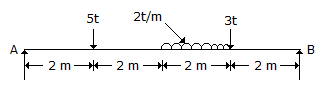# Civil Engineering - Applied Mechanics

### Exercise :: Applied Mechanics - Section 2

6.

A body is said to move with Simple Harmonic Motion if its acceleration, is

 A. always directed away from the centre, the point of reference B. proportional to the square of the distance from the point of reference C. proportional to the distance from the point of reference and directed towards it D. inversely proportion to the distance from the point of reference E. none of these.

Explanation:

No answer description available for this question. Let us discuss.

7.

One end of a light string 4 m in length is fixed to a point on a smooth wall and the other end fastened to a point on the surface of a smooth sphere of diameter 2.25 m and of weight 100 kg. The reaction between the sphere and the wall of the arrangement made is

 A. 102.5 kg B. 105.5 kg C. 108.5 kg D. 110 kg.

Explanation:

No answer description available for this question. Let us discuss.

8.

Which one of the following laws is not applicable to a simple pendulum ?.

 A. The time period does not depend on its magnitude B. The time period is proportional to its length l C. The time period is proportional to l where l is length D. The time period is inversely proportional to g where g is the acceleration due to gravity.

Explanation:

No answer description available for this question. Let us discuss.

9.

The ratio of the reactions RA and RB of a simply supported beam shown in below figure isA. 0.5 B. 0.4 C. 0.67 D. 1 E. 1.5

Explanation:

No answer description available for this question. Let us discuss.

10.

Effect of a force on a body depends upon its

 A. direction B. magnitude C. position D. all the above.